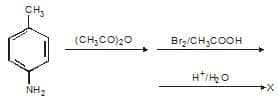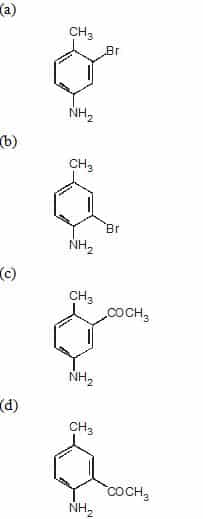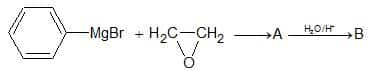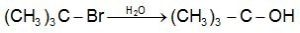# AIIMS MBBS Chemistry Question and Answer50 Multiple choice AIIMS Chemistry Question based on AIIMS MBBS Entrance Exam.

Question: Mixture of sand and sulphur may best be separated by
(a) Fractional crystallisation from aqueous solution
(b) Magnetic method
(c) Fractional distillation
(d) Dissolving in CS2 and filtering

Ans. (d)

Question: An organic compound containing C, H and O contains 52.5% C and 13.04% Hr Vapour density of the
compound is 23. Its molecular formula will be :
(a) C2H6O
(b) C3H8O
(c) C4H8O
(d) C5H10O

Ans. (a)

Question: The species that does not contain peroxide ion is
(a) PbO2
(b) H2O2
(c) SrO2
(d) BaO2

Ans. (a)

Question: What are the shapes of (i) C1F3 and (ii) BrF3 molecules ?
(a) Both are planar trigonal
(b) Both have distorted T-shaped
(c) (i) is planar trigonal and (ii) is T-shaped
(d) (i) is T-shaped and (ii) is planar trigonal

Ans. (b)

Question: Irrespective of the source, pure sample of water always yields 88.89% mass of oxygen and 11.11% mass of hydrogen. This is explained by the law of
(a) Conservation of mass
(b) Constant composition
(c) Multiple proportions
(d) Constant volume

Ans. (b)

Question: Which of the following salts is colourless ?
(a) CdCI2
(b) CuSO4-5H2O
(c) MnSO4-7H2O
(d) NiSO4-7H

Ans. (a)

Question: Zinc sulphate contains 22.65% of zinc and 43.9% of water of crystallization. If the law of constant proportions is true, then the weight of zinc required to produce 20 g of the crystals will be
(a) 45.3 g
(b) 4.53 g
(c) 0.453 g
(d) 453 g

Ans. (b)

Question: Boiling point of the following compounds follow the order.
(a) CH3CH3 <CH3NH2 <CH3OH< HCOOH
(b) CH3NH2 < CH3OH < CH3CH3 < HCOOH
(c) CH3OH < CH3CH3 < CH3NH2 < HCOOH
(d) HCOOH < CH3NH2 < CH3OH < CH3CH3

Ans. (a)

Question: The number of optical isomers of an organic compound having n asymmetric carbon atoms will be
(a) 2n + 1
(b) n2
(c) 2n
(d) 2n – 1

Ans. (c)

Question:Which of the following will have the maximum dipole moment ?
(a) CH3F
(b) CH3CI
(c) CH3Br
(d) CH3I

Ans. (b)

Related: hybridization practice worksheet

Question: Atoms consists of protons, neutrons and electrons. If the mass of neutrons and electrons were made half and two  times  respectively to their actual masses, then the atomic mass of 6C12
(a) Will remain approximately the same
(b) Will become approximately two  times
(c) Will remain approximately half
(d) Will be reduced by 25%

Ans. (d)

Question: Borax structure contains:
(a) two BO4 groups and two BO3 groups
(b) four BO4 groups only
(c) four BO3 groups only
(d) three BO4 and one BO3 groups

Ans. (a)

Question: The electronic configuration of a dipositive metal M2+ is 2, 8, 14 and its atomic weight is 56 a.m.u. The number of neutrons in its nuclei would be
(a) 30
(b) 32
(c) 34
(d) 42

Ans. (a)

Question: Which of the following is neutral refractory material?
(a) SiO2
(b) MgO
(c) CaO
(d) SiC

Ans. (d)

Question: O22– is the symbol of ….. ion
(a) Oxide
(b) Superoxide
(c) Peroxide
(d) Monoxide

Ans. (c)

Question: Which of the following is not correct for D2O ?
(a) BVP is higher than H2O
(c) Viscosity is higher than H2O at 25°
(b) D2O reacts slowly than H2O
(d) Solubility of NaCI in it is more than H2O

Ans. (d)

Ques. One mole of NaCl (s) on melting absorbed 30.5 kJ of heat and its entropy is increased by 28.8 JK–1. The melting point of NaCl is
(a) 1059 K
(b) 30.5 K
(c) 28.8 K
(d) 28800 K

Ans. (a)

Ques. Which one of the following is not a property of hydrophilic solutions?
(a) High concentrations of dispersed phase can be easily attained
(b) Coagulation is reversible
(c) Viscosity and surface tension are about the same as for water
(d) The charge of the particle depends on the   values of the medium; it may be positive, negative or even zero

Ans. (c)

Ques. Temperature of maximum density in H2O and D2O respectively are
(a) 277.15 K, 284.75 K
(b) 273.15 K, 277.15 K
(c) 277.15 K, 285.75 K
(d) 284.75 K, 277.15 K

Ans. (a)

Question: If ethylene, carbon monoxide and water is heated at high temperature, which of the following is formed
(a) C4H8O2
(b) C2H5COOH
(c) CH3COOH
(d) CH2 = CH – COOH

Ans. (b)

Question: An ester (A) with molecular formula C9Hi0O2 was treated with excess of CH3MgBr and the compound so formed was treated with cone. H2SO4 to form olefin (B). Ozonolysis of B gave ketone with formula C8H8O. Which shows positive iodoform test the structure of A is:
(a) CH3CH2COC6CH5
(b) C6H5COOC2H5
(c) C6HSCOOC6H5
(d) CH3COC6H4COCH3

Ans. (b)

Related: mcq on fungi

Question: A 0.2 molal aqueous solution of a weak acid (HX) is 20% ionised. The freezing point of this solution is (Given Kf = 1.86o C/m for water)
(a) –0.31oC
(b)–0.45oC
(c) –0.53oC
(d) –090oC

Ans. (b)

Question:The density of H2S (mol. wt. 34) at 27°C and 2 atm pressure (R = 0.0821 atm/mol K):
(a) 2.76g/L
(b) 27.6 g/L
(c) 2.76 mg/L
(d) none of these

Ans. (a)

Question: The pyknometric density of sodium chloride crystal is 2.165 x 103 kgm–3 while its X-rays density is 2.178 x 103 kgm–3. The fraction of unoccupied sites in sodium chloride crystal is
(a) 5.96 x 10–3
(b) 5.96
(c) 5.96 x 10–2
(d) 5.96 x 10–1

Ans. (a)

Question: In which compound 8 : 8 coordination is found
(a) CsCl
(b) MgO
(c) Al2O3
(d) All of these

Ans. (a)

Question: Which of the following reactions with H2S does not produce metallic sulphide
(a) ZnCl2
(b) CdCl2
(c) COCl2
(d) CuCl2

Ans. (c)

Question: At what temperature will the average speed of CH4 molecules have the same value as O2 has at 300 K
(a) 1200 K
(b) 150 K
(c) 600 K
(d) 300 K

Ans. (b)

Question: In amines, the hybridisation state of N is
(a) sp
(b) sp2
(c) sp3
(d) sp2d

Ans. (c)

Related: work power problems worksheet

Question: Which of the following is not an electrophile
(a) Cl+
(b) Na+
(c) H+
(d) BF3

Ans. (b)

Question: In an experiment during the analysis of a carbon compound, 145 l of H2 was collected at 760 mm Hg pressure and 27oC temperature. The mass of H2 is nearly
(a) 10 g
(b) 12 g
(c) 24 g
(d) 6 g

Ans. (b)

Question: Which is the decreasing order of stability?
(i) CH– CH – CH3
(ii) CH– CH – O – CH3
(iii) CH3CH – CO – CH3
(a) (i) < (ii) < (iii)
(b) (i) > (ii) > (iii)
(c) (iii) > (i) > (ii)
(d) (ii) > (iii) > (i)

Ans. (b)

Question: The order of reactivities of methyl halides in the formation of Grignard reagent is
(a) CH3I > CH3Br > CH3Cl
(b) CH3Cl > CH3Br > CH3I
(c) CH3Br > CH3Cl > CH3I
(d) CH3Br > CH3I > CH3Cl

Ans. (a)

Question: Half-life of radium is 1580 yrs. Its average life will be
(a) 2.5 x 103 yrs
(b) 1.832 x 103 yrs
(c) 2.275 x 103 yrs
(d) 8.825 x 102 yrs

Ans. (c)

Question: When D-glucose is oxidized by Ag:0 gives:
(a) L-glucaric acid
(b) D-glucaric acid
(c) D-gluconic acid
(d) L-gluconic acid

Ans. (c)

Question: The dissociation constant of two acids HA1 and HA2 are 3.14 x 10–4 and 1.96 x 10–5 respectively. The relative strength of the acids will be approximately
(a) 1 : 4
(b) 4 : 1
(c) 1 : 16
(d) 16 : 1

Ans. (b)

Question:The product is :Ans. (b)

Question: In the sequence of reactions,The product B is

(a) Benzyl alcohol
(b) 2-Phenyl ethanol
(c) 1-Phenyl ethanol
(d) Quinol

Ans. (b)

Question: Which ion has the lowest radius from the following ions
(a) Na+
(b) Mg2+
(c) Al3+
(d) Si4+

Ans. (d)

Question: In amines, the hybridisation state of N is
(a) sp
(b) sp2
(c) sp3
(d) sp2d

Ans. (c)

Question: When Na reacts with liquid NH3 the following substance is formed:
(a) [Na(NH3)x]
(b) [e(NH3)y]
(c) NaNH2
(d) NaxNH3y

Ans. (b)

Question: To calculate the amount of work done in joules during reversible isothermal expansion of an ideal gas, the volume must be expressed in
(a) m3 only
(b) dm3 only
(c) cm3 only
(d) Any one of them

Ans. (d)

Question: 1 mole of N2O4 (g) at 300 K is kept in a closed container under one atmosphere. It is heated to 600 K when 20% by mass of N2O4 (g) decomposes to NO2 (g). The resultant pressure is:
(a) 1.2 atm
(b) 2.4 atm
(c) 2.0 atm
(d) 1.0 atm

Ans. (b)

Question: The oxidation number of sulphur in S8, S2F2, H2S respectively, are
(a) 0, +1 and – 2
(b) + 2, +1 and – 2
(c) 0, + 1 and + 2
(d) – 2, + 1 and – 2

Ans. (a)

Question: Mark the gas which turns lime water milky
(a) H2S
(b) SO2
(c) Cl2
(d) CO2

Ans. (b), (d)

Question: The ion which exhibits green colour?
(a) Cu2+
(b) Mn2+
(c) Co2+
(d) Ni2+

Ans. (d)

Question: Which of the following statements is correct for CsBr3
(a) It is a covalent compound
(b) It contains Cs3+ and Br ions
(c) It contains Cs+ and Br3ions
(d) It contains Cs+, Brand lattice Br2 molecule

Ans. (c)

Ques. Which is the strongest reducing agent?
(a) F
(b) Cl
(c) Br
(d) I

Ans. (d)

Question: A sudden large jump between the values of second and third ionisation energies of an element would be associated with the electronic configuration
(a) 1s2, 2s2 p6, 3s1
(b) 1s2, 2s2 p6, 3s2 p1
(c) 1s2, 2s2 p6, 3s2p2
(d) 1s2, 2s2 p6, 3s2

Ans. (d)

Question: A hydride of nitrogen which is acidic is
(a) NH3
(b) N2H2
(c) N3H
(d) N2H4

Ans. (c)

Question: Which element having following electronic configurations has minimum ionization potential
(a) 1s1
(b) 1s2, 2s2 2p6
(c) 1s2, 2s2 2p6, 3s1
(d) 1s2, 2s2 2p2

Ans. (c)

Ques. The following reaction belongs to(a) Elimination reaction
(b) Substitution reaction
(d) Displacement reaction

Ans. (b)

Ques. The most stable conformation of n-butane is
(a) Skew boat
(b) Eclipsed
(c) Gauche
(d) Staggered

Ans. (d)

Ques. The process used for the removal hardness of water is
(a) Calgon
(b) Baeyer
(c) Serpeck
(d) Hoope

Ans. (a)

Question: If the H+ concentration is decreased from 1M to 10-4 M at 25o C for the couple MnO4/Mn2+, then the oxidizing power of the MnO4/Mn2+ couple decrease by :
(a) -0.18 V
(b) 0.18 V
(c) 0.38 V
(d) -0.38 V

Ans. (c)

Ques. Maximum number of electrons in any orbit is
(a) n2
(b) 2n2
(c) ½ n2
(d) None of these

Ans. (b)

Question: HCl is added to following oxides. Which one would give H2O2
(a) MnO2
(b) PbO2
(c) BaO
(d) None of these

Ans. (d)

Ques. Which of the following electrolytes is least effective in coagulating a colloidal solution of ferric hydroxide
(a) K3[Fe(CN)6]
(b) K2CrO4
(c) KBr
(d) K2SO4

Ans. (c)

Question: Which of the following pair will not produce dihydrogen gas
(a) Cu + HCl (dil.)
(b) Fe + H2SO4
(c) Mg + steam
(d) Na + alcohol

Ans. (a)

Ques. A gem dihalide is not the product of the following reactions
(a) Vinyl chloride + HCl
(b) Acetone + PCl5
(c) Methylacetylene + 2HBr
(d) Ethyne +2Cl2

Ans. (d)

Related: mental health awareness quiz

(a) Soda glass
(b) Jena glass
(c) Pyrex glass
(d) Flint glass

Ans. (d)

Question: If the concentration of CrO4ions in a saturated solution of silver chromate is 2 x 10–4. Solubility product of silver chromate will be
(a) 4 x 10–8
(b) 8 x 10–12
(c) 12 x 10–12
(d) 32 x 10–12

Ans. (d)

Question: BaSO4 and carbon on heating reacts to produce
(a) Ba + SO2 + CO2
(b) BaS + CO
(c) BaS + O2 + SO2
(d) BaCO3 + S + O2

Ans. (b)

Question: The atomic radii of alkali metals (M) lie in the order Li < Na < K < Rb but the radii of M+ ions in aqueous solution lie in the reverse order Li+ > Na+ > K+ > Rb+. What is the reason for this reverse order (on going from Li to Rb) ?
(a) Gradual increase in ionisation energy
(b) Increasing weakness of the metallic bond
(c) Increasing electropositive character
(d) Decreasing degree of hydration

Ans. (d)

Question: Transition metal with low oxidation number will act as
(a) A base
(b) An acid
(c) An oxidising agent
(d) None of these

Ans. (c)

Question: Which of the following pair will have effective magnetic moment equal
(a) Cr+3 and Mn+2
(b) Cr+2 and Fe+2
(c) V+2 and Sc+3
(d) Ti+2 and V+2

Ans. (b)

Question: Which is least soluble in water
(a) AgCl
(b) AgBr
(c) AgI
(d) Ag2S

Ans. (d)

Question: Which one of the following has the maximum number of unpaired electrons
(a) Mg2+
(b) Ti3+
(c) V3+
(d) Fe2+

Ans. (d)

Question: The rate law for a reaction between the substances A and B is given by, rate = k[A]n [B]m. On doubling the concentration of A and halving the concentration of B, the ratio of the new rate to the earlier rate of the reaction will be as
(a) ½(m + n)
(b) (m + n)
(c) (n – m)
(d) 2(n – m)

Ans. (d)

Question: The coordination number and oxidation state of Cr in K3 [Cr(C2O4)3] are, respectively
(a) 4 and +2
(b) 6 and +3
(c) 3 and +3
(d) 3 and 0

Ans. (b)

Related: herb trivia quiz

Question: Mixture is heated with dil. H2SO4 and the lead acetate paper turns black by the evolved gases. The mixture contains
(a) Sulphite
(b) Sulphide
(c) Sulphate
(d) Thiosulphate

Ans. (b)

Question: Total number of isomers of C6H14 are
(a) 4
(b) 5
(c) 6
(d) 7

Ans. (b)

Question: The hybridization state of carbon atoms in the product formed by the reaction of ethyl chloride with aqueous potassium hydroxide is
(a) sp
(b) sp2
(c) sp3
(d) sp3d

Ans. (c)

Question: The chief impurity present in red bauxite is :
(a) SiO2
(b) Fe2O3
(c) K2SO4
(d) NaF

Ans. (b)

Ques. Preparation of alkyl halides in laboratory is least preferred by
(a) Halide exchange
(b) Direct halogenation of alkanes
(c) Treatment of alcohols
(d) Addition of hydrogen halides to alkenes

Ans. (b)

Ques. The freezing point of a solution containing 4.8 g of a compound in 60 g of benzene is 4.48. What is the molar mass of the compound (Kf = 5.1 km–1), (freezing point of  benzene = 5.5o)
(a) 100
(b) 200
(c) 300
(d) 400

Ans. (d)

Ques. Buna-s rubber is which of the following of 1-3-butadiene and styrene
(a) Polymers
(b) Copolymer
(d) Condensation polymer

Ans. (b)

Ques. Which of the following is a protein?
(a) Pepsin
(c) ATP
(d) Glutamin

Ans. (a)

Related: questions on binomial expansion

Ques. Which of the following tests can be used for the distinction of ethanol and ethanol
[A] Pdoform test
[B] Lucas test
[C] Victor Meyer test
[D] Copper coil test
(a) A and D
(b) A and B
(c) C and D
(d) Only A

Ans. (a)

Ques. The correct order of second ionization potential of carbon, nitrogen, oxygen and fluorine is
(a) C > N > O > F
(b) O > N > F > C
(c) O > F > N > C
(d) F > O > N > C

Ans. (c)

Ques. Which of the following is the mechanism of the reaction, which takes place on fusing nitrobenzene with solid caustic potash?
(a) Electrophilic substitution
(d) Nucleophilic substitution

Ans. (d)

Ques. Most compact complexation ABC, ABC — exhibits the following
(a) FCC
(b) BCC
(c) HCP
(d) None of the above

Ans. (a)